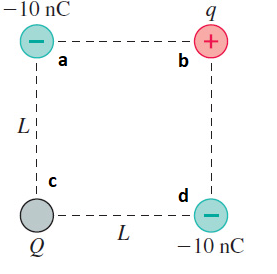# Problem: (Figure 1) shows four charges at the corners of a square side L.What magnitude and sign of charge Q will make the force on charge q zero?

###### FREE Expert Solution
95% (303 ratings)
###### FREE Expert Solution

Forces involving electric charges is given by:

$\overline{){\mathbf{F}}{\mathbf{=}}\frac{{\mathbf{kq}}_{\mathbf{1}}{\mathbf{q}}_{\mathbf{2}}}{{\mathbf{r}}^{\mathbf{2}}}}$

Summing perpendicular vectors:

$\overline{){\mathbf{F}}{\mathbf{=}}\sqrt{{{\mathbf{F}}_{\mathbf{x}}}^{\mathbf{2}}\mathbf{+}{{\mathbf{F}}_{\mathbf{y}}}^{\mathbf{2}}}}$

95% (303 ratings)###### Problem Details

(Figure 1) shows four charges at the corners of a square side L.
What magnitude and sign of charge Q will make the force on charge q zero?Frequently Asked Questions

What scientific concept do you need to know in order to solve this problem?

Our tutors have indicated that to solve this problem you will need to apply the Coulomb's Law (Electric Force) concept. You can view video lessons to learn Coulomb's Law (Electric Force). Or if you need more Coulomb's Law (Electric Force) practice, you can also practice Coulomb's Law (Electric Force) practice problems.

What professor is this problem relevant for?

Based on our data, we think this problem is relevant for Professor Krishna & Weatherford's class at UF.